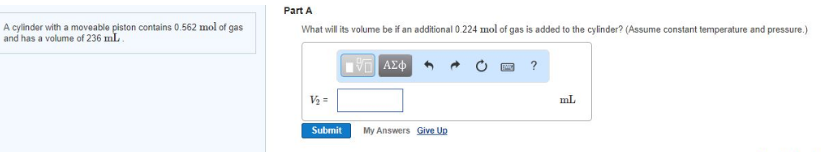Chemistry Practice Problems Chemistry Gas Laws Practice Problems Solution: A cylinder with a moveable piston contains 0.562 m...

# Solution: A cylinder with a moveable piston contains 0.562 mol of gas and has a volume of 236 mL. Part AWhat will its volume be if an additional 0.224 mol of gas is added to the cylinder? (Assume constant temperature and pressure.)

###### Problem

A cylinder with a moveable piston contains 0.562 mol of gas and has a volume of 236 mL.

Part A

What will its volume be if an additional 0.224 mol of gas is added to the cylinder? (Assume constant temperature and pressure.)View Complete Written Solution

Chemistry Gas Laws

Chemistry Gas Laws

#### Q. The following picture represents a sample of gas at a pressure of 1 atm, a volume of 1 L, and a temperature of 25°C. (Figure 1)What would happen to th...

Solved • Tue Jul 24 2018 14:27:42 GMT-0400 (EDT)

Chemistry Gas Laws

#### Q. Part AA syringe containing 1.63 mL of oxygen gas is cooled from 96.4°C to 0.1°C. What is the final volume Vf of oxygen gas? (Assume that the pressure ...

Solved • Tue Jul 24 2018 13:48:42 GMT-0400 (EDT)

Chemistry Gas Laws

#### Q. What pressure would need to be applied to sample of gas with an initial volume of 979.2 mL at a pressure of 588.0 torr to decrease the volume to 325.2...

Solved • Tue Jul 24 2018 13:24:12 GMT-0400 (EDT)

Chemistry Gas Laws

#### Q. A balloon containing methane gas has a volume of 5.06 L at 95.0°C. What volume will the ballon occupy at 1.90 × 102°C?

Solved • Tue Jul 24 2018 13:17:00 GMT-0400 (EDT)